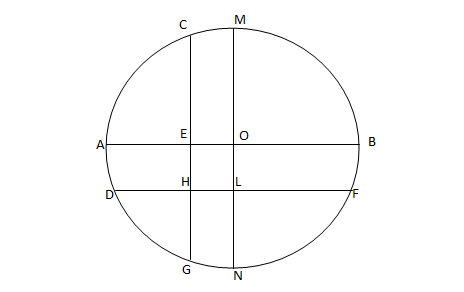Question 22

# In the following figure, the diameter of the circle is 3 cm. AB and MN are two diameters such that MN is perpendicular to AB. In addition, CG is perpendicular to AB such that AE:EB = 1:2, and DF is perpendicular to MN such that NL:LM = 1:2. The length of DH in cm isSolution

Let EO = x, So, AE = 1.5 - x
AE : EB = 1:2 => x = 1/2

(1.5-x):(1.5+x) = 1:2.

x=0.5.

So, EO = 0.5
Similarly, OL = 0.5
Now, EOLH is a parallelogram and EO = OL = 0.5
In triangle DOL, DO = radius = 1.5 and OL = 0.5
So, DL = $$\sqrt2$$
=> DH = $$(2\sqrt2 - 1)/2$$